## Solutions of Refraction of Light Lakhmir Singh Manjit Kaur LAQ, MCQ, HOTS, and VSAQ Pg No. 240 Class 10 Physics

29A. With the help of a labeled diagram explain how a convex lens converges a beam of parallel light rays.

→ According to the laws of refraction, when a beam of light rays parallel to one another and also to the principal axis of the convex lens fall on the lens, the incident rays pass through the lens, gets refracted and is focused on focus.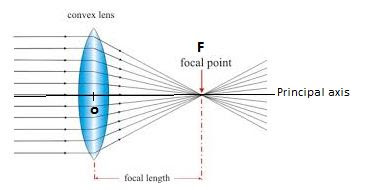Mark the principal axis, optical centre, principal focus and focal length of the convex lens on the diagram.

29B. State whether convex lens has a real focus or a virtual focus.

→ Real focus.

29C. List some things that convex lens and concave mirror have in common.

→ Parallel rays of light coming from infinity converges at the focus.

30A. With the help of a labeled diagram, explain how a concave lens diverges a beam of parallel light rays.
Mark the principal axis, optical centre, principal focus and focal length of the concave lens on the diagram.According to the laws of refraction, When a beam of light rays parallel to one another and also to the principal axis of the concave lens fall on the lens, the incident rays pass through the lens, gets refracted and when these diverging rays are produced backwards they appear to meet at a point F (focus) on the left side of the lens.

30B. State whether concave lens has a real focus or a virtual focus.

→ Virtual focus.

30C. List some things that concave lens and convex mirror have in common.

→ Parallel rays of light coming from infinity diverges at focus.

31. Draw ray diagrams to represent the nature, position and relative size of the image formed by a convex lens for the object placed:
(a) At 2F1
(b) Between F1 and the optical centre 0 of the lens.
Which of the above two cases shows the use of convex lens as a magnifying glass? Give reasons for your choice.

→ (a) Object at 2F1 :(b) Object between F1 and the optical centre O of the lens :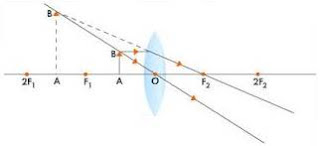The case 2 shows the use of convex lens as a magnifying glass as the image formed is erect and magnified.

32A. An object is placed well outside the principal focus of a convex lens. Draw a ray diagram to show how the image is formed, and say whether the image is real or virtual.32B. What is the effect on the size and position of the image of moving the object?
(i) towards the lens, and
(II) Away from the lens?

→ (i) the image size will keep on increasing if object is moved towards the lens, till the object reaches focus. After that, the size decreases but the image remains magnified.
(ii) If object is moved away from the lens, the size will keep on decreasing and the image keeps on shifting towards the lens.

33A. Explain what is meant by a virtual, magnified image.

→ A virtual magnified image cannot be taken on a screen and whose size is larger than that of the object.

33B. Draw a ray diagram to show the formation of a virtual magnified image of an object by a convex lens. In your diagram, the position of object and image with respect to the principal focus should be shown clearly.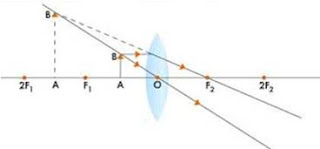33C. Three convex lenses are available having focal lengths of 4 cm, 40 cm and 4 m respectively. Which one would you choose as a magnifying glass and why?

→ Convex lens having 4 cm focal length should be chosen because it will produce greatest magnification.

34A. Explain why, a real image can be projected on a screen but a virtual image cannot.

→ A real image is formed when rays actually meet and in a virtual image, light rays appear to meet at a point when produced backwards (but do not actually meet) after refraction through a lens.

34B. Draw a ray diagram to show the formation of a real diminished image of an object by a convex lens. In your diagram, the position of object and image with respect to the principal focus should be shown clearly.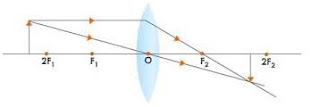34C. Name one simple optical instrument in which the above arrangement of convex lens is used.

→ A simple camera is a simple optical instrument in which the above arrangement of convex lens is used.

Multiple Choice Questions (MCQs)-Pg-241

35. A convex lens has a focal length of 10 cm. At which of the following position should an object be placed so that this convex lens may act as a magnifying glass?
A. 15 cm
B. 7 cm
C. 20 cm
D. 25 cm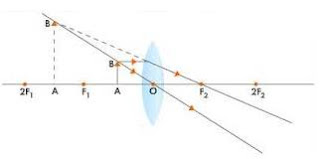36. Which one of the following materials cannot be used to make a lens?
A. Water
B. Glass
C. Plastic
D. Clay

→ Water, glass and plastic reflect light, but clay does not as it is opaque.

37. A small bulb is placed at the focal point of a converging lens. When the bulb is switched on, the lens produces:
A. A convergent beam of light
B. A divergent beam of light
C. A parallel beam of light
D. A patch of coloured light38. An illuminated object is placed at a distance of 20 cm from a converging lens of focal length 15 cm. The image obtained on the screen is:
A. Upright and magnified
B. Inverted and magnified
C. Inverted and diminished
D. Upright and diminished39. An object is placed between f and 2f of a convex lens. Which of the following statements correctly describes its image?
A. Real, larger than the object
B. Erect, smaller than the object
C. Inverted, same size as object
D. Virtual, larger than the object40. Which of the following can make a parallel beam of light when light from a bulb falls on it?
A. Concave mirror as well as concave lens
B. convex mirror as well as convex lens
C. Concave mirror as well as convex lens
D. convex mirror as well as concave lens

→ Concave mirror as well as convex lens makes a parallel beam of light when light from a bulb falls on it.

41. In order to obtain a real image twice the size of the object with a convex lens of focal length 15 cm, the object distance should be:
A. More than 5 cm but less than 10 cm
B. More than 10 cm but less than 15 cm
C. More than 15 cm but less than 30 cm
D. More than 30 cm but less than 60 cm42. A converging lens is used to produce an image of an object on a screen. What change is needed for the image to be formed nearer to the lens?
A. Increase the focal length of the lens
B. Insert a diverging lens between the lens and the screen
C. Increase the distance of the object from the lens
D. Move the object closer to the lensIn all the other cases image formed is real, inverted and magnified.

43. A convex lens of focal length 8 cm forms a real image of the same size as the object. The distance between object and its image will be:
A. 8 cm
B. 16 cm
C. 24 cm
D. 32 cm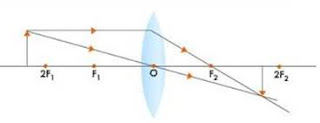44. A virtual, erect and magnified image of an object is to be obtained with a convex lens. For this purpose, the object should be placed:
A. Between 2F and infinity
B. Between F and 2F
C. Between F and optical center
D. At F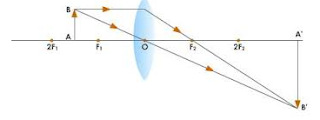45. A burning candle whose flame is 1.5 cm tall is placed at a certain distance in front of a convex lens. An image of candle flame is received on a white screen kept behind the lens. The image of flame also measures 1.5 cm. If f is the focal length of convex lens, the candle is placed:
A. At f
B. Between f and 2 f
C. At 2 f
D. Beyond 2f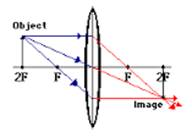Questions Based on High Order Thinking Skills (HOTS)-Pg-242

46. A lens of focal length 15 cm forms an erect image three times the size of the object. The distance between the object and image is:
(a) 20 cm
(b) 16 cm
(c) 24 cm
(d) 36 cm

→ (a)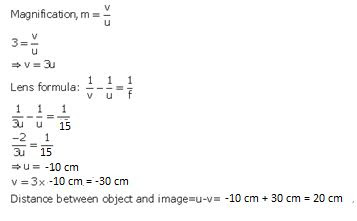47. If an object is placed 23 cm from a converging lens, the image formed is slightly smaller than the object. If the object is placed 21 cm from the lens, the image formed is slightly larger than the object. The approximate focal length of the lens is:
(a) 11 cm (b) 10 cm
(c) 18 cm (d) 20 cm

→ (a) 11 cm
The image is slightly smaller than the object when the object lies beyond 2f; and the image is slightly larger than the object when the object between f and 2f. This means that between 21 cm and 23 cm lies 2f. Out of the given options, 22 cm lies between 21 cm and 23 cm.
So, 2f = 22 cm
f = 11 cm.

48. Object is placed at the following distances from a convex lens of focal length 16 cm:
(a) 35 cm (b) 32 cm
(c) 20 cm (d) 10 cm
Which position of the object will produce?
(I) a magnified real image?
(ii) A magnified virtual image?
(iii) A diminished real image?
(iv) An image of same size as the object?

→ Here, f=16 cm and 2f=32cm
(i) 20 cm (Because a magnified real image is formed when the object is placed between f and 2f)
(ii) 10 cm (Because a magnified virtual image is formed when the object is placed between f and the lens)
(iii) 35 cm (Because a diminished real image is formed when the object is placed beyond 2f)
(iv) 32 cm (Because an image of same size as the object is formed when the object is placed at 2f).

49. When an object is placed at a distance of 40 cm from a convex lens, an image of the same size as the object is formed. What will be the nature of image formed when the object is placed at a distance of?
(a) 10 cm from the lens?
(b) 20 cm from the lens?

→ Here, 2f = 34 cm, f = 17 cm
(a) When the object is placed at a distance of 10 cm from the lens, the object lies within the focus. Hence, the image formed is virtual, erect and magnified.
(b) When the object is placed at a distance of 20 cm from the lens, the object lies between f and 2f. Hence, the image formed is real, inverted and magnified.

50A. Draw a diagram to show how a converging lens focusses parallel rays of light.

→ A diagram to show how a converging lens focusses parallel rays of light.’50B. How would you alter the above diagram to show how a converging lens can produce a beam of parallel rays of light?

→ We can alter the above diagram to show how a converging lens can produce a beam of parallel rays of light by Placing a source of light at the focus of the converging lens.1. Write the formula for a lens connecting image distance (), object distance (u) and the focal length (f). How does the lens formula differ from the mirror formula?

→ The formula for a lens connecting image distance (), object distance (u) and the focal length (f) isThis is also known as the lens formula.
Mirror formula is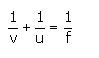The lens formula has a minus sign (-) between 1/v and 1/u whereas the mirror formula has a plus sign (+) between 1/v and 1/u.

2. Write down the magnification formula for a lens in terms of object distance and image distance. How does this magnification formula for a lens differ from the corresponding formula for a mirror?

→ Magnification (m) formula for a lens in terms of object distance (u) and image distance (v) is: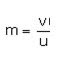Magnification formula for a mirror in terms of object distance (u) and image distance (v) is: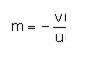Magnification formula for a mirror has a minus sign (-) but the magnification formula for a lens has no minus sign.

3. What is the nature of the image formed by a convex lens if the magnification produced by the lens is +3?

→ Virtual and erect is the nature of the image formed by a convex lens if the magnification produced by the lens is +3.

4. What is the nature of the image formed by a convex lens if the magnification produced by the lens is, - 0.5?

→ Real and inverted is the nature of the image formed by a convex lens if the magnification produced by the lens is, - 0.5.

5. What is the position of image when an object is placed at a distance of 12 cm from a convex lens of focal length 12 cm?

→ u = -12 cm, f = 12 cm
We have6. Describe the nature of image formed when an object is placed at a distance of 30 cm from a convex lens of focal length 15 cm.

→ The nature of image formed when an object is placed at a distance of 30 cm from a convex lens of focal length 15 cm is real and inverted.

7. At what distance from a converging lens of focal length 14 cm must an object be placed in order that an image of magnification 1 will be produced?8. State and explain the New Cartesian Sign Convention for spherical lenses.

→ Following sign convention is used for measuring various distances during the formation of images by lenses:
(ii) The distances measured in the same direction as that of incident light are taken as positive.
(iii) The distances measured against the direction of incident light are taken as negative.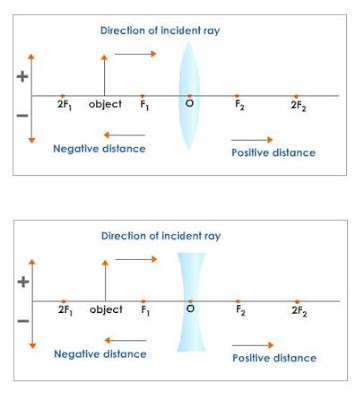● All distances on the principal axis are measured from the optical center.
● The distances measured in the direction of incident rays are positive and all the distances measured in the direction opposite to that of the incident rays are negative.

● All distances measured above the principal axis are positive. Thus, height of an object and that of an erect image are positive and all distances measured below the principal axis are negative.

9. An object 4 cm high is placed at a distance of 10 cm from a convex lens of focal length 20 cm. Find the position, nature and size of the image. Draw the diagram to represent the position.The diagram to represent the position is10. A small object is so placed in front of a convex lens of 5 cm focal length that a virtual image is formed at a distance of 20 cm. Find the magnification.11. Find the position and nature of the image of an object 8 cm high and 10 cm in front of a convex lens of focal length 6 cm.

→ Height of image = 8cm.Image is formed 15 cm behind the mirror and the image formed is real and inverted.

12. Calculate the focal length of a convex lens which produces a virtual image at a distance of 60 cm of an object placed 20 cm in front of it.13. An object is placed at a distance of 100 cm from a converging lens of focal length 50 cm.
(i) What is the nature of image?
(ii) What is the position of image?

→ (i) Real and inverted
(ii) v = 50 cm; The image is formed 50 cm behind the convex lens (on its right side).14. A convex lens produces an inverted image magnified three times of an object placed at a distance of 15 from it. Calculate the image distance.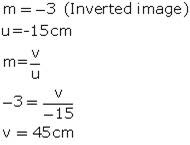15. A converging lens of focal length 10 cm is placed at a distance of 20 cm from a screen. How far from the lens should an object be placed so as to form its real image on the screen?16. An object 10 cm high is held 25 cm away from a converging lens of focal length 10 cm. find the position, size and nature of the image formed. Also draw the ray diagram.17. At what distance should an object be placed from a convex lens of focal length 12 cm to obtain an image at 24 cm from it on the other side? What will be the magnification produced in this case?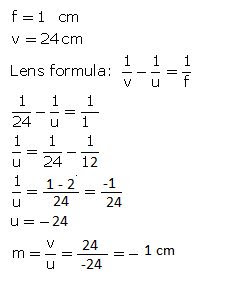18. An object 3 cm tall is placed on the axis of a convex lens of focal length 5 cm at a distance of 10 m from the optical center of the lens. Find the nature, position and size of the image formed. Which case of image formation by convex lenses is illustrated by this example?

→ Real and inverted;
v = + 5 cm ; The image is formed 5 cm behind the convex lens ; 0.01 cm
h1= 2 cm
f = 5 cm
u = –10 m = –1000 cmv = 5.02 cm
The image is formed 5.02 cm behind the convex lens and is real and inverted.
Since the object distance is much greater than the focal length, this example illustrates the case when the object is placed at infinity.

19. The filament of a lamp is 80 cm from a screen and a converging lens forms an image of it on a screen, magnified three times. Find the distance of the lens from the filament and the focal length of the lens.

→ Given:
The filament of a lamp is 80 cm from a screen, T = -80 cm Magnification, m = 3
Formula used:
Magnification of the lens, m = v/u
Where v is the image distance from the lens u is the object distance from the lensThe distance of filament from the screen, T= Sum of the distance between the lens and filament(u) and the distance between the lens and the image(v)From the above statement T = u + v Putting the value in the above equation, we get 80 cm = 4u object distance, u =-20 cm
Thus, the distance of the lens from the filament = -20 cm ;
The distance of the lens from the screen is 60 cm
Using the lens formula,Where v is the image distance u is the object distance f is the focal length Putting the values in the above relation, we get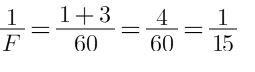f = + 15 cm
Thus, the focal length of the convex lens is +15 cm

20. An erect image 2.0 cm high is formed 12 cm from a lens, the object being 0.5 cm high. Find the focal length of the lens.

→ Height of image = 2 cm
Image distance = 12 cm
Height of object= 0.5 cm
M = height of image/ height of object = 2/0.5 =- 4
M = v/u
-4 = 12/u
u =- 3 cm
using lens formula,f = + 4.0 cm.

21. A convex lens of focal length 0.10 misused to form a magnified image of an object of height 5 m placed at a distance of 0.08 m from the lens. Calculate the position, nature and size of the image.

→ f = 0.10 m
m = 5 m
object distance (u) = 0.08 m
using the lens formula,v = - 0.40 m ;
The position of image is 0.40 m from the lens on the same side as the object
(on the left of lens);
The nature of image is virtual and erect; The size of image is 25 m.

22. A convex lens of focal length 6 cm is held 4 cm from a newspaper which has print 0.5 cm high. By calculation, determine the size and nature of the image produced.

→ Focal length = 6 cm
Object distance = -4 cm
Height of object = 0.5 cm
Using lens formula,Size of the image = 1.5 cm high;
The nature of the image formed is Virtual, erect and magnified (3 times).

23. Determine how far an object must be placed in front of a converging lens of focal length 15 cm in order to produce an erect (upright) image of linear magnification 4.

→ f = 10 cm
m = +4 (upright image)v = 4u
Lens formula: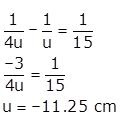The object must be placed 11.25 in front of the converging lens.

24. A lens of focal length 20 cm is used to produce a ten times magnified image of a film slide on a screen. How far must the slide be placed from the lens ?

→ Focal length = 20 cmfrom above we get:-
V = -10 u (From cross multiplying)
Using the lens formula,Putting the values in above equation we get:-Hence the slide should be placed 220 cm behind the lens.
the slide be placed 220 cm behind the lens.

25. An object placed 4 cm in front of a converging lens produces a real image 12 cm from the lens.
(a) What is the magnification of the image?
(b) What is the focal length of the lens?
(c) Draw a ray diagram to show the formation of
Image. Mark clearly F and 2F in the diagram.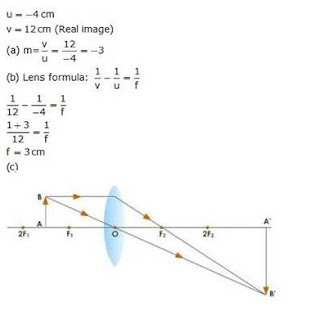26A. An object 2 cm tall stands on the principal axis of a converging lens of focal length 8 cm. Find the position, nature and size of the image formed if the object is:
(i) 12 cm from the lens
(ii) 6 cm from the lens

→ Height of object = 2 cm
Focal length = 8 cm
(i) Object distance (u)=12 cm
Using lens formula,Image distance (v) = + 24 cm
The image is 24 cm behind the lens ; The nature of the image formed is Real and inverted ;
Height of image = 4 cm
(ii) Object distance (u)= 6 cm
Using lens formula,Image distance (v) = - 24 cm ; The image is 24 cm in front of the lens ; The nature of the image formed is Virtual and erect ;

Height of image = 8 cm

26B. State one practical application each of the use of such a lens with the object in position (i) and (ii).

→ (i) practical application of the use of such a lens with the object in position (i) is film projector
(ii) practical application of the use of such a lens with the object in position (i) is Used as a magnifying glass.

27A. An object 3 cm high is placed 24 cm away from a convex lens of focal length 8 cm. Find by calculations, the position, height and nature of the image.

→ Height of object = 3 cm
Object distance = -24 cm
Using lens formulaImage distance (v) = + 12 cm ; The image is formed 12 cm behind the lens; 1.5 cm high; The nature of the image is Real and inverted.

27B. If the object is moved to a point only 3 cm away from the lens, what are the new position, height and nature of the image?

→ Height of object = 3 cm
Object distance = -3 cm
Using lens formulaImage distance (v) =- 4.8 cm; The image is formed 4.8 cm in front of the lens (on its left side); 4.8 cm high ; The nature of the image is Virtual and erect.

27C. Which of the above two cases illustrates the working of a magnifying glass?

→ Case b illustrates the working of a magnifying glass.

28A. Find the nature, position and magnification of the images formed by a convex lens of focal length 0.20 m if the object is placed at a distance of :
(i) 0.50 m (ii) 0.25 m (iii) 0.15 m

→ focal length = 0.20 m

(i) Object distance = 0.50 m
Using lens formula,Image distance, v = + 0.33 m ; The image is formed 0.33 m behind the lens ; m = - 0.66 ; Real and inverted.

(ii) Object distance = 0.25 m
Using lens formula,Image distance, v = + 1.00 m ; The image is formed 1.00 m behind the lens ; m = - 4.0 ; Real and inverted.

(iii) Object distance = 0.15 m
Using lens formula,Image distance, v = - 0.60 m ; The image is formed 0.60 m in front of the lens ; m = + 4.0; Virtual and erect.

28B. Which of the above cases represents the use of convex lens in a film projector, in a camera, and as a magnifying glass?